# Decimal Number Lines

Print Rate 0 stars Common Core
Lesson size:
Message preview:
Someone you know has shared lesson with you:

To play this lesson, click on the link below:

https://www.turtlediary.com/lesson/decimal-number-lines.html

Hope you have a good experience with this site and recommend to your friends too.

Login to rate activities and track progress.
Login to rate activities and track progress.

A number line is a visual way to see how numbers relate to each other. Just as the name sounds, a number line places numbers, evenly spaced, on a horizontal line and each point on the line corresponds to a number. Just like on a number line with positive integers (the counting numbers), the decimal numbers increase the farther to the right we move.

Let's compare a whole number line to a decimal number line.

Notice, how on a number line involving whole numbers with positive integers, the digits get bigger as we move to the right, just like how we count (1, 2, 3)On a decimal number line involving tenths, there are 10 evenly spaced segments to represent each consecutive tenth. The decimal digits increase until we reach the next whole number (1).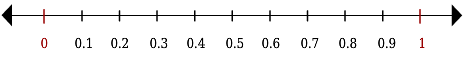So if we start with zero and begin counting the parts of whole as tenths, we count each tenth until the next whole number (1): one tenth, two tenths, three tenths, four tenths, nine tenths, one whole.

What happens after we reach one whole (1)?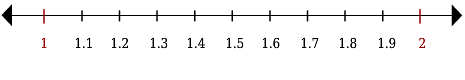Then, we keep counting one whole (1) plus each additional tenth: one and one tenth, one and two tenths, one and three tenths, one and nine tenths, two.

And when we reach 2, which is the next whole number? The same pattern applies.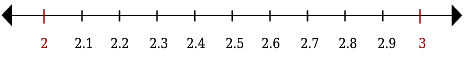Next, let's look at when happens when a decimal number line involves hundredths.

Again, there are 10 evenly spaced segments to represent each consecutive hundredth. If we start at zero (0) and begin counting parts of the whole as hundredths, we count each hundredth until we get to one tenth (0.1): one hundredth, two hundredths, three hundredths nine hundredths, ten hundredths (0.10), which we simplify to one tenth (0.1).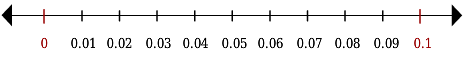Remember, one tenth (0.1) is equal to ten hundredth (0.10).

What happens when we reach 0.1?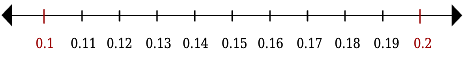Just keep counting hundredths until twenty hundredths (0.20), which is equivalent to two tenths (0.2).

And when we reach 0.2? Yes, the same pattern applies.A decimal number line allows us to see how counting decimals is very similar to counting positive integers.

Let's practice using decimal number lines.

## 1) Complete the number line with decimal numbers with the place value of tenths.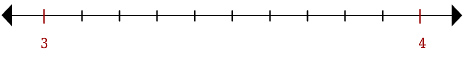Beginning with the whole number 3, count on from 3 by tenths filling in each consecutive tenth underneath the horizontal line.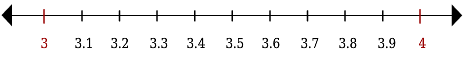## 2) Complete the number line with decimal numbers with the place value of tenths.Beginning with the whole number 5 count on from 5 by tenths filling in each consecutive tenth underneath the horizontal line.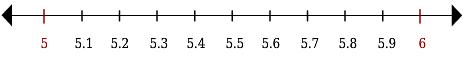## 3) Label the missing decimal numbers on the number line.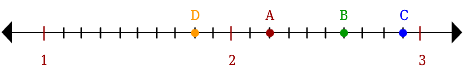Beginning with the whole number 1 count on one tenth to identify the decimal number of point D. Then, beginning with the whole number 2, count on from 2 by tenths to identify point A, B and C.

A = 2.2

B = 2.6

C = 2.9

D = 1.8

## 4) Label the missing decimal numbers on the number line.Beginning with the whole number 7 count on one tenth to identify the decimal number of point C. Then, beginning with the whole number 8, count on from 8 by tenths to identify point B, A and D.

A = 8.7

B = 8.2

C = 7.1

D = 8.8

## 5) Complete the number line with decimal numbers with the place value of hundredths.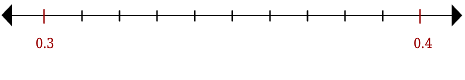Beginning with the decimal number 0.3, count on from 0.3 by hundredths filling in each consecutive hundredth underneath the horizontal line.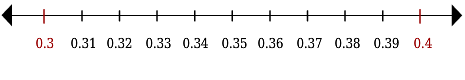## 6) Complete the number line with decimal numbers with the place value of hundredths.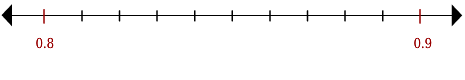Beginning with the decimal number 0.8, count on from 0.8 by hundredths filling in each consecutive hundredth underneath the horizontal line.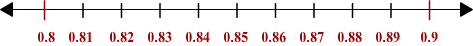## Decimal Number Lines

• A number line is a visual way to see how numbers relate to each other.
• Just like on a number line with positive integers (the counting numbers), the decimal numbers increase the farther to the right we move.
• On a decimal number line involving tenths, there are 10 evenly spaced segments to represent each consecutive tenth. The decimal's digits increase until we reach the next whole number and the pattern repeats.
• On a decimal number line involving hundredths, there are 10 evenly spaced segments to represent each consecutive hundredth. If we begin counting parts of the whole as hundredths, we count each hundredth until we get to the next tenth.

## Similar Lessons

Become premium member to get unlimited access.